# 计算统计学与数据科学 05：重抽样方法

## 墨尔本大学 MAST90083 课程笔记

Posted by YEY on August 31, 2020

# Lecture 05 重抽样方法

• Gareth, J., Daniela, W., Trevor, H., & Robert, T. (2013). An intruduction to statistical learning: with applications in R. Spinger.
• Hastie, T., Tibshirani, R., & Friedman, J. (2009). The elements of statistical learning: data mining, inference, and prediction. Spinger Science & Business Media.

## 1. 交叉验证法

### 1.1 训练误差 vs. 测试误差

• 测试误差 是使用某种统计学习方法预测在一个新的观测 (即在训练模型时没有用到的一个观测) 上的响应值所产生的平均误差。

• 相反，通过将统计学习方法用于训练观测上，可以轻松计算出 训练误差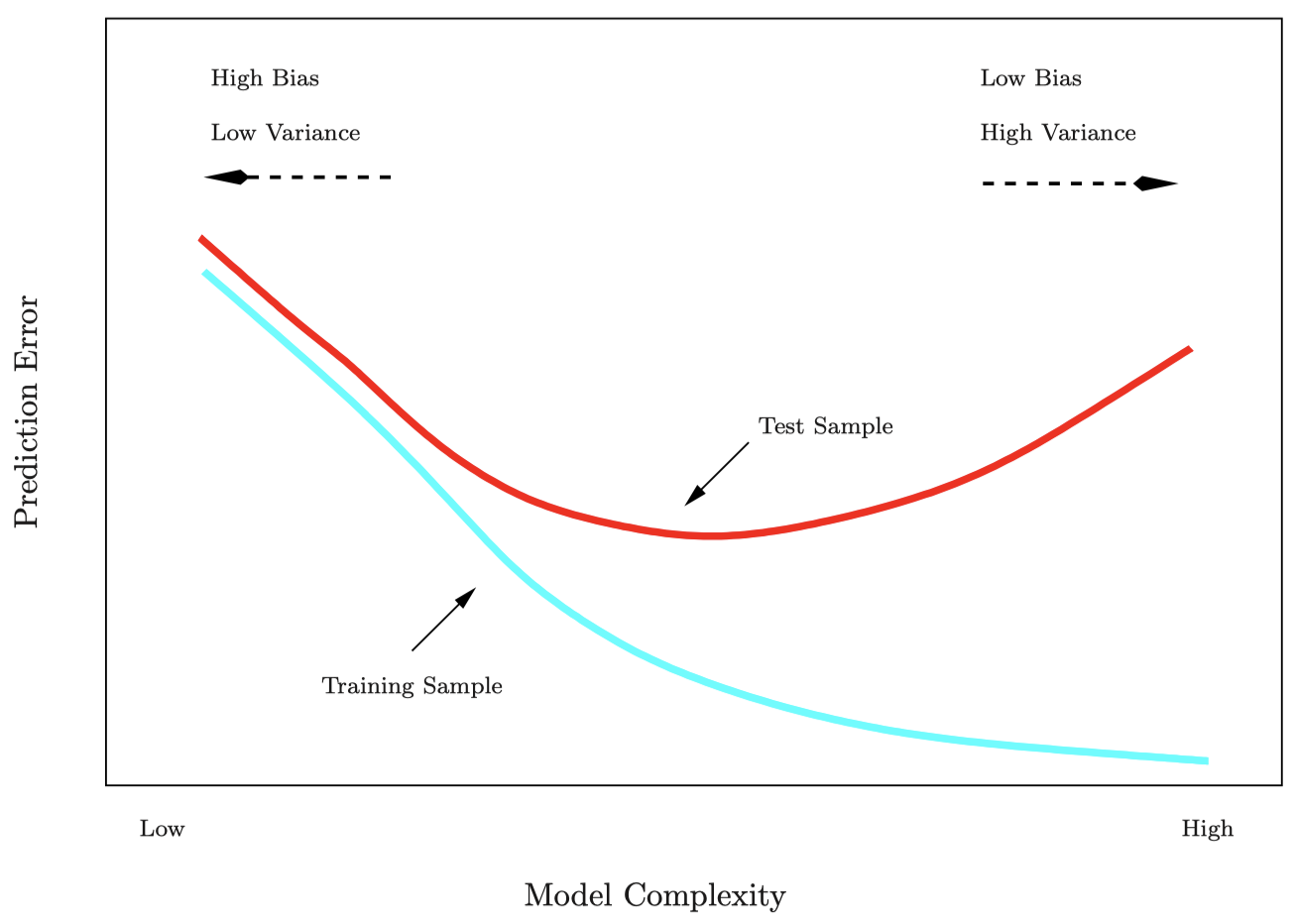### 1.2 验证集方法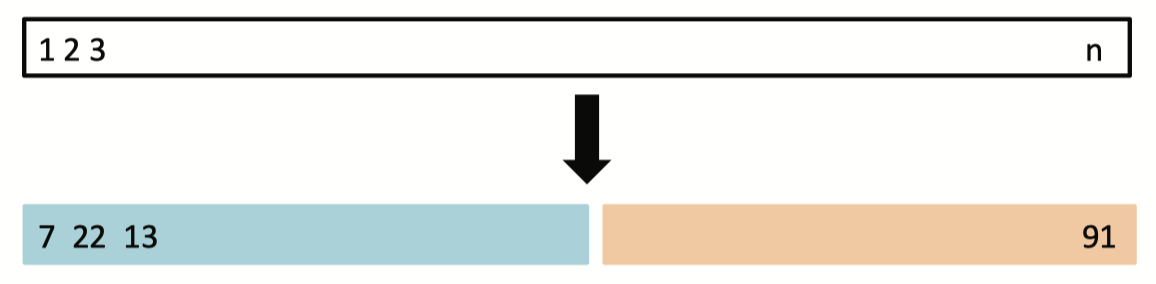#### 例子：汽车数据集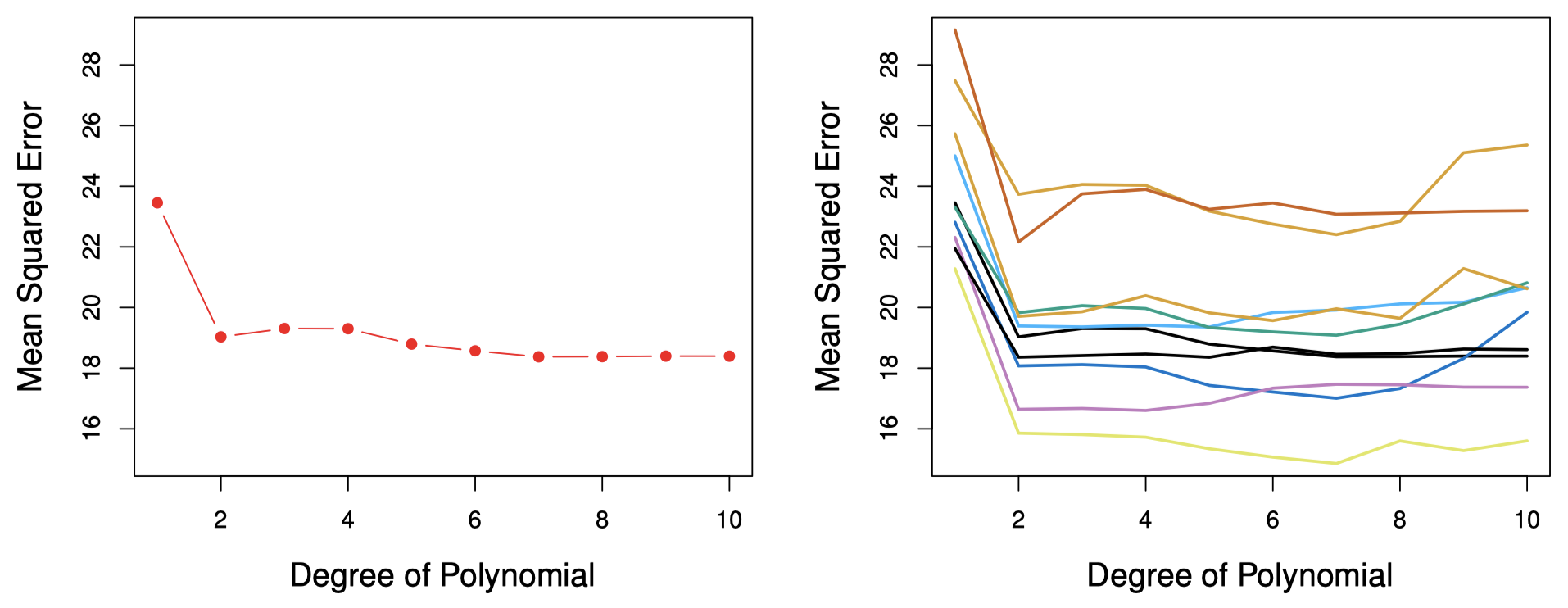#### 验证集方法的缺点

1. 测试误差的验证集法估计的 波动很大 (如图 3 右图所示)，这取决于具体哪些观测被包括在训练集中，哪些观测被包括在验证集中。

2. 在验证集方法中，只有一部分观测 (即那些被包含在训练集而非验证集中的观测) 被用于拟合模型。由于训练样本越少，统计学习方法的表现越差，这意味着，验证集误差可能会 高估 在整个数据集上拟合模型所得到的测试误差。

### 1.3 $K$-折交叉验证

$K$-折交叉验证 是一种广泛用于估计测试误差的方法。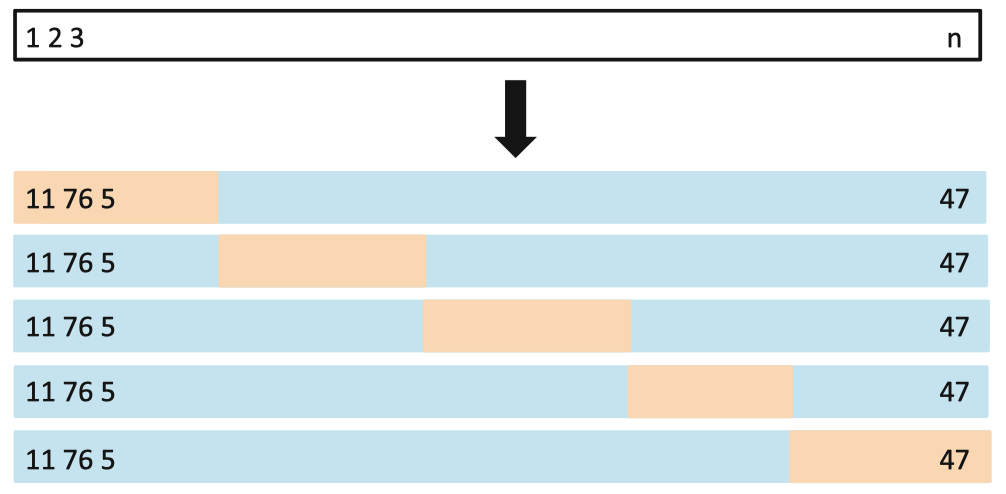$\mathrm{CV}_{(K)}=\sum_{k=1}^{K}\dfrac{n_k}{n} \mathrm{MSE}_k$

#### 留一交叉验证

LOOCV 方法的计算量可能很大，因为模型需要被拟合 $n$ 次。但是，使用最小二乘法来拟合线性或多项式回归时，可以将 LOOCV 的计算成本缩减到与单个模型拟合成本相同。计算公式如下：

$\mathrm{CV}_{(n)}=\dfrac{1}{n}\sum_{i=1}^{n}\left(\dfrac{y_i - \hat y_i}{1- h_i}\right)^2$

LOOCV 有时很有用，但通常会使 数据重组不够充分 (即每次只留出一个观测作为验证集，可能导致每次训练集之间的差异过小)。由于每折观测的估计值高度相关，因此它们的平均值可能具有很高的方差。

#### $K$-折 CV vs. LOOCV

Auto 数据集上分别用 LOOCV 和 $10$-折 CV 方法，得到用 horsepower 的多项式函数拟合线性回归模型，来预测 mpg 所产生的测试集均方误差的一个估计，如图 5 所示。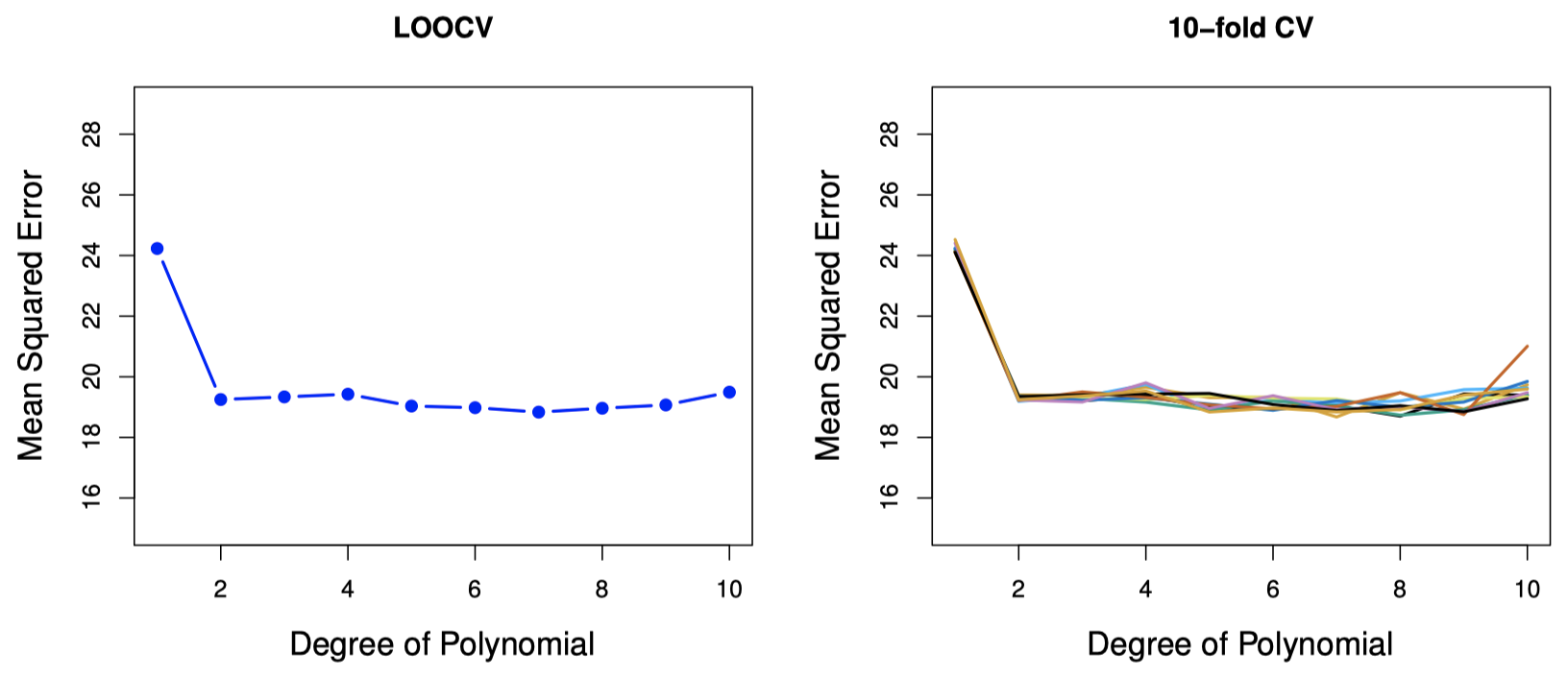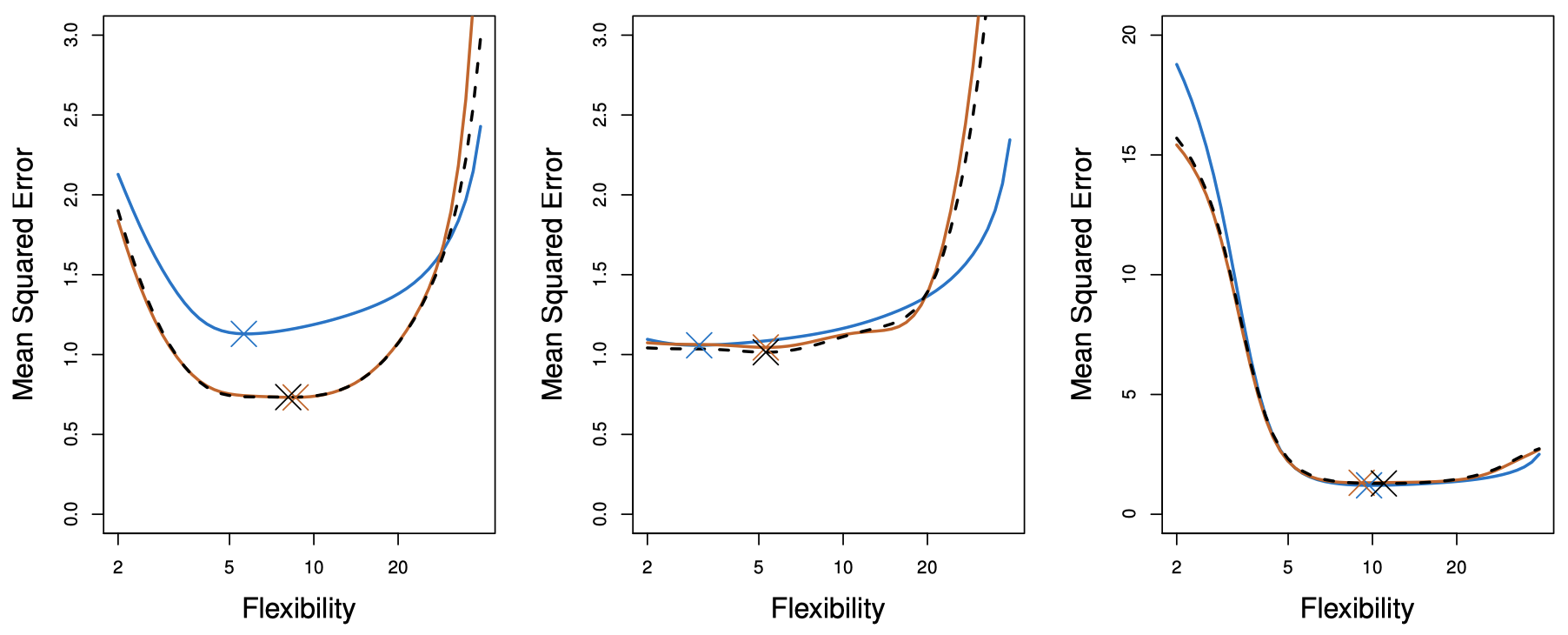### 1.4 交叉验证法的其他问题

$K = 5$ 或 $10$ 为这种偏差-方差权衡提供了一个很好的折衷方案。

### 1.5 交叉验证法用于分类问题

$\mathrm{CV}_{(K)} = \sum_{k=1}^{K}\dfrac{n_k}{n}\text{Err}_k$

$\mathrm{CV}_{(K)}$ 的标准差的估计为：

$\widehat{\mathrm{SE}}(\mathrm{CV}_{(K)}) = \sqrt{\sum_{k=1}^{K}(\text{Err}_k-\overline{\text{Err}_k})^2 / (K-1)}$

### 1.6 交叉验证法：正确与错误做法

1. 从 $5000$ 个预测变量和 $50$ 个样本开始，找到与类别标签相关性最大的 $100$ 个预测变量。
2. 然后，我们应用一个分类器 (例如，逻辑回归)，并且仅使用前一步得到的 $100$ 个预测变量。

• 错误做法：交叉验证仅应用于步骤 2。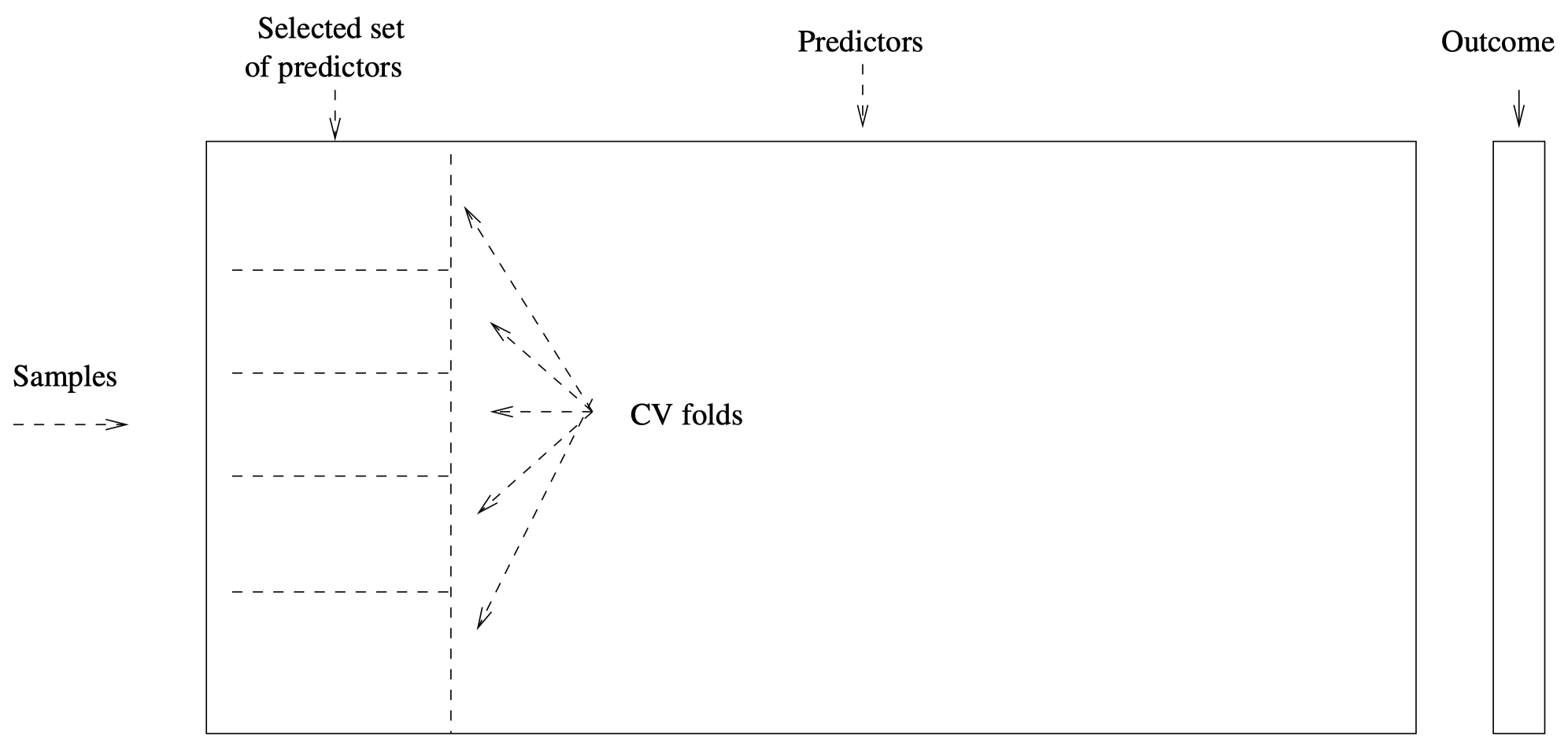图 7：交叉验证法的错误使用方式。

• 正确做法：交叉验证同时应用于步骤 1 和步骤 2。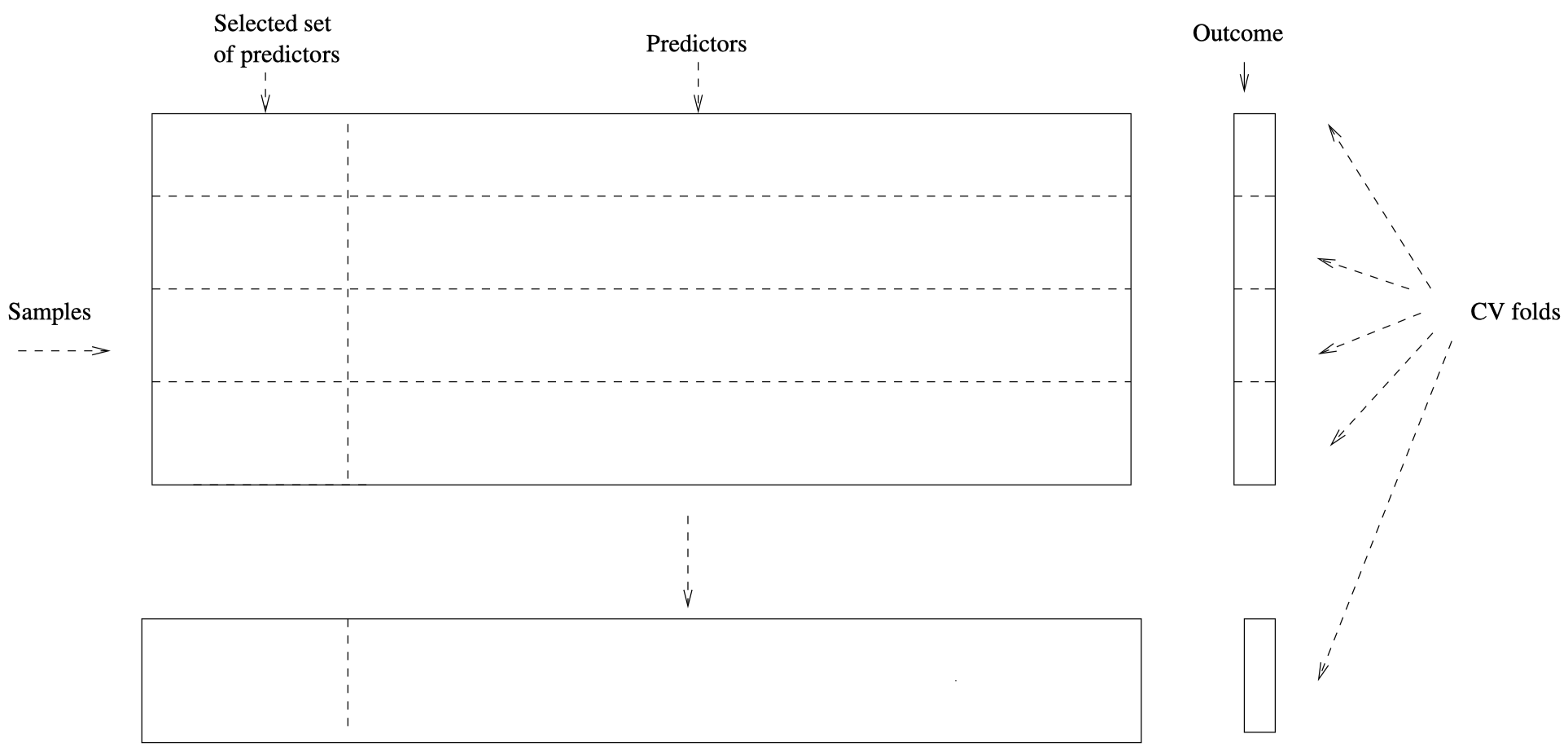图 8：交叉验证法的正确使用方式。

## 2. Bootstrap 方法

Bootstrap 是一种灵活而强大的统计工具，可以用它来衡量一个指定的估计器或统计学习方法中的不确定因素。

### 2.1 Bootstrap 名称的由来

Bootstrap 一词的使用源于用自己的 靴子 (bootstraps) 来帮助自己站起来，这被广泛认为是基于 18 世纪 Rudolph Erich Raspe 的小说《蒙克豪森男爵的惊人历险记》中的某个场景：

### 2.2 一个简单的例子

$\alpha = \dfrac{\sigma_Y^2 - \sigma_{XY}}{\sigma_X^2 + \sigma_Y^2 -2\sigma_{XY}}$

$\hat \alpha = \dfrac{\hat \sigma_Y^2 - \hat \sigma_{XY}}{\hat \sigma_X^2 + \hat \sigma_Y^2 -2\hat \sigma_{XY}}$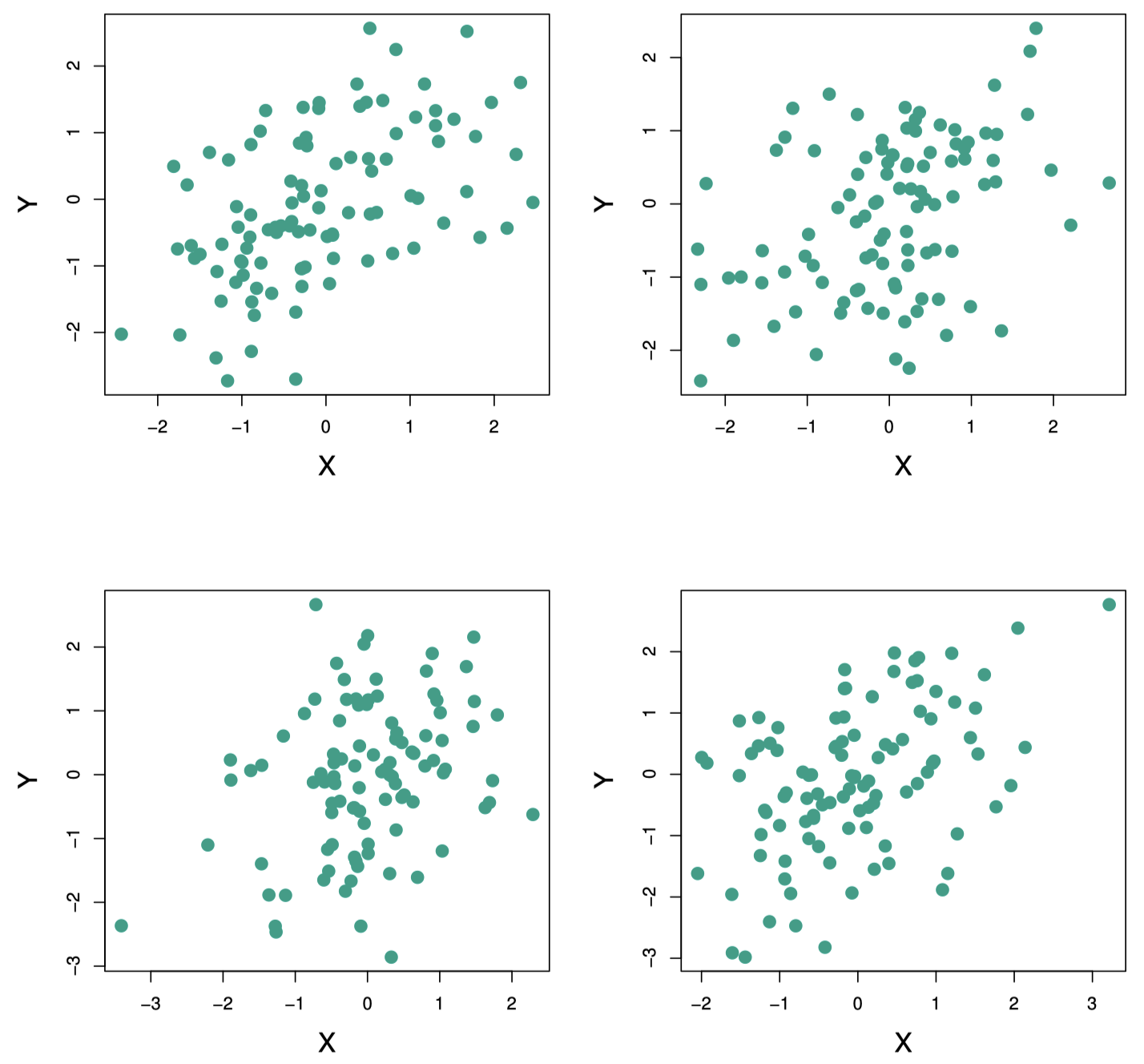$\alpha$ 全部 $1000$ 个估计的均值为：

$\overline \alpha = \dfrac{1}{1000}\sum_{r=1}^{1000}\hat \alpha_r = 0.5996$

$\sqrt{\dfrac{1}{1000-1}\sum_{r=1}^{1000}(\hat \alpha_r - \overline \alpha)^2} =0.083$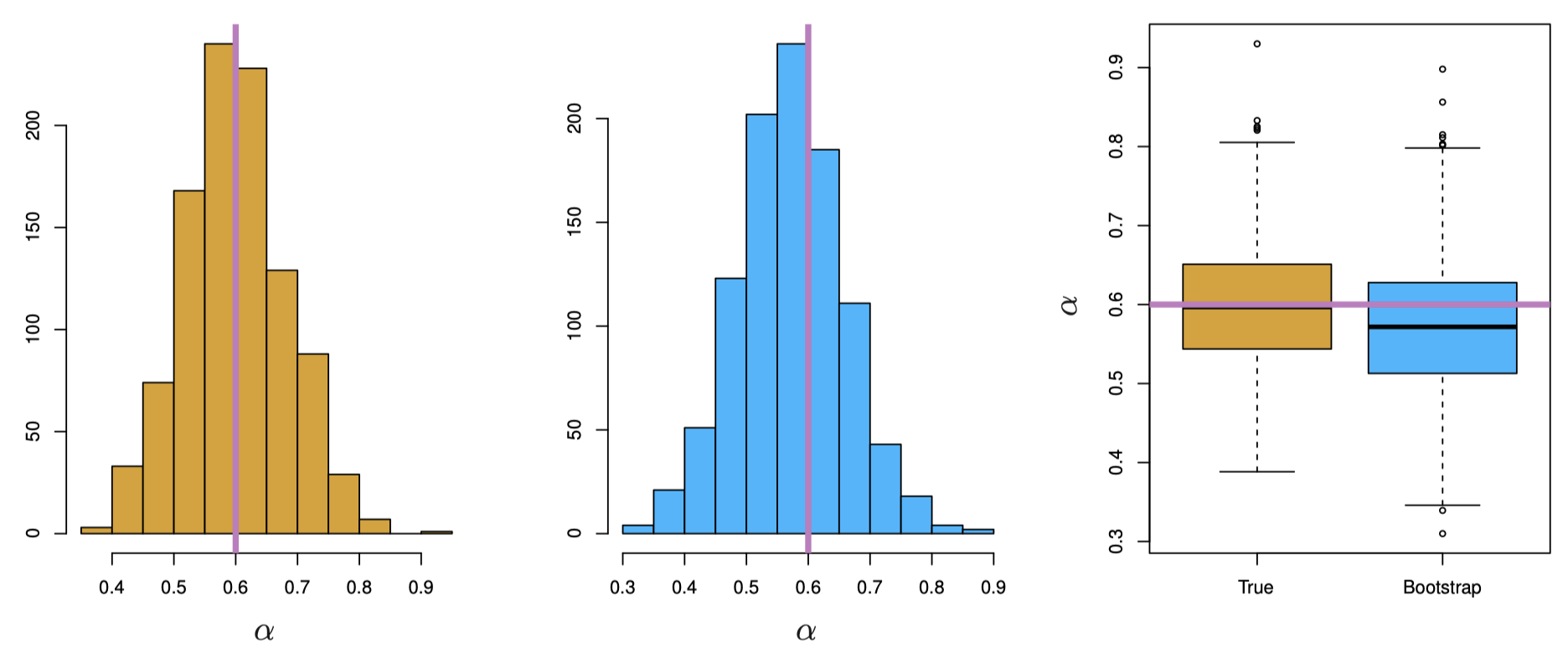### 2.4 Bootstrap 方法的例子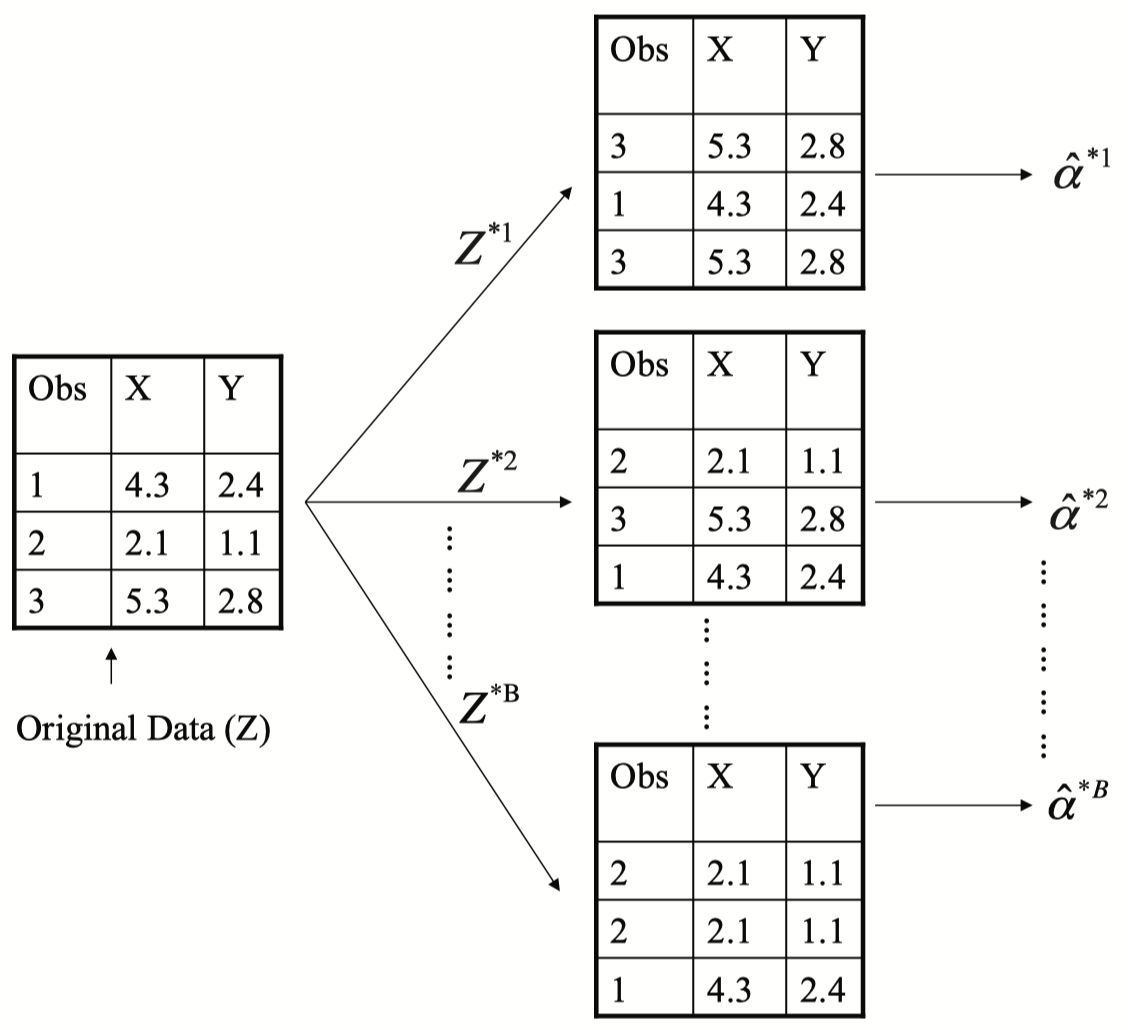$\mathrm{SE}_B(\hat \alpha)=\sqrt{\dfrac{1}{B-1}\sum_{r=1}^{B}(\hat \alpha^{*r} - \overline{\hat \alpha^*})^2}$

### 2.5 一般的 bootstrap 方法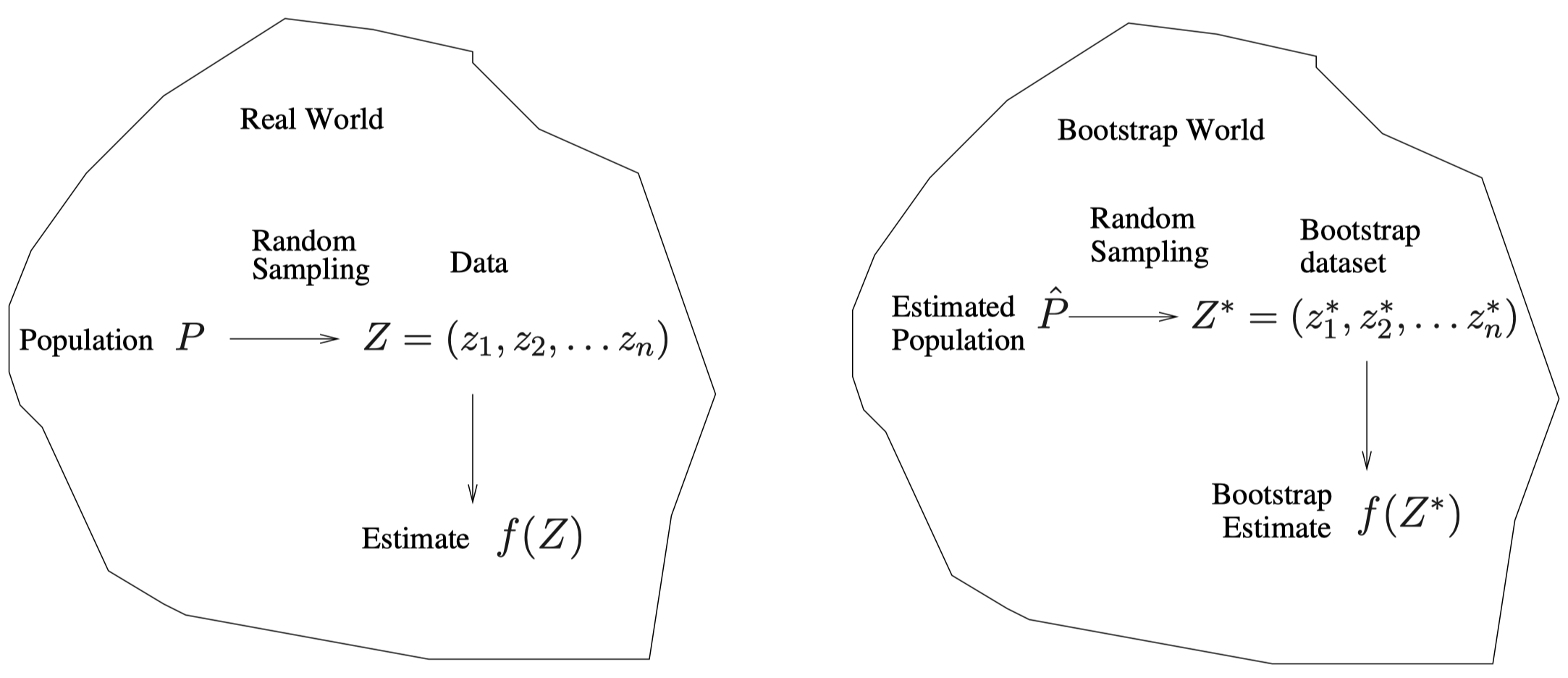### 2.6 Bootstrap 的一些其他用法

Bootstrap 方法主要用于获得某个估计的 标准误 (standard errors)

Bootstrap 方法还为我们提供了总体参数的一个 近似置信区间。例如，在图 10 的中图所示的直方图中，可以看到，$1000$ 个 $\alpha$ 估计值的 $5\%$ 和 $95\%$ 分位数分别为 $0.43$ 和 $0.72$。因此，该方法为我们提供了真实 $\alpha$ 的一个近似 $90\%$ 置信区间 $[0.43, 0.72]$，我们称之为 Bootstrap 百分比置信区间，这也是通过 bootstrap 获得置信区间的众多方法中最简单的一种。

• 在交叉验证中，$K$ 个验证折里的每一个都与其他 $K-1$ 个用于训练的折不同：不存在重叠。而这也是交叉验证法成功的关键。

• 为了使用 bootstrap 估计预测误差，我们可以考虑将每个 bootstrap 数据集用作训练样本，并将原始样本用作验证样本。

• 但是，每个 bootstrap 样本都与原始数据有很大的 重叠 部分。事实上，大约有三分之二的原始数据点会出现在每个 bootstrap 样本中。简单证明如下：

$\Pr\{i\text{-th observation} \in Z^{*b}\} = 1-\left(1- \dfrac{1}{n}\right)^n \approx 1- e^{-1} =0.632$
• 这将导致 bootstrap 严重低估 真实的预测误差。因为大量数据重叠导致过拟合使得 bootstrap 的估计误差仅为真实误差的大约三分之一。

• 而另一方面，将原始样本作为训练样本，将 bootstrap 数据集作为验证样本，结果更糟糕。

#### 消除重叠

• 通过仅对那些没有 (偶然) 出现在当前 bootstrap 样本中的观测进行预测，可以部分解决此问题。
• 但是，这种方法非常复杂。因此，交叉验证为估计预测误差提供了一种更简单、更具吸引力的方法。

### 2.7 预验证

#### 预验证的例子

Van’t Veer 等人的论文 (Nature, 2002) 中提供了关于这个问题的一个例子：他们的微阵列数据具有 $78$ 个病例上测量的 $4918$ 个基因，这些基因来自乳腺癌研究。其中，预后良好组包含 $44$ 例，预后不良组包含 $34$ 例。“微阵列” 预测变量的构成如下：

1. 选择 $70$ 个基因，它们与上面 $78$ 个类标签具有最大的绝对相关性的。
2. 利用这 $70$ 个基因，构建了一个最近质心分类器 $C(x)$。
3. 将分类器应用于 $78$ 个微阵列，得到每个病例 $i$ 的二分预测变量 $z_i=C(x_i)$。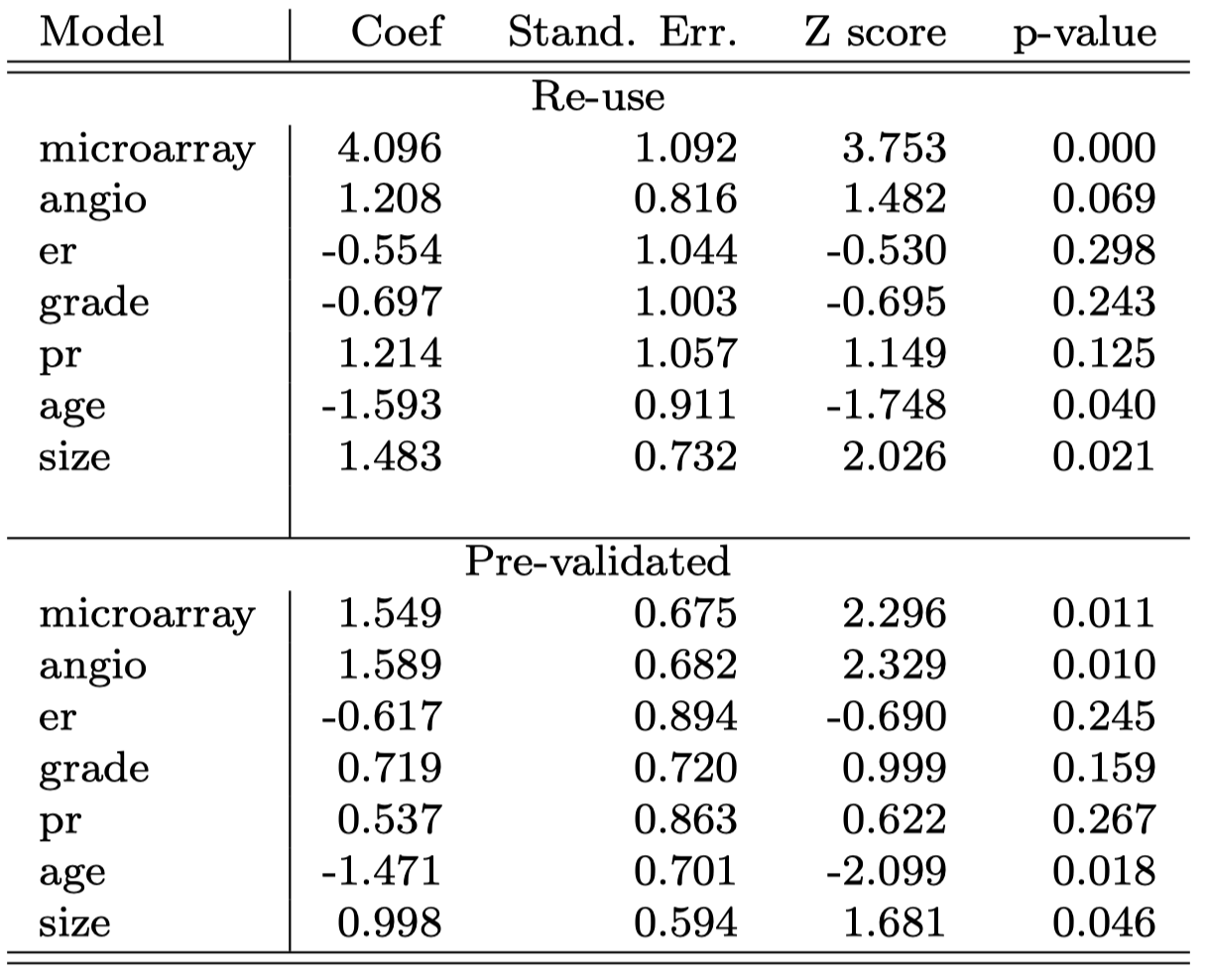#### 预验证流程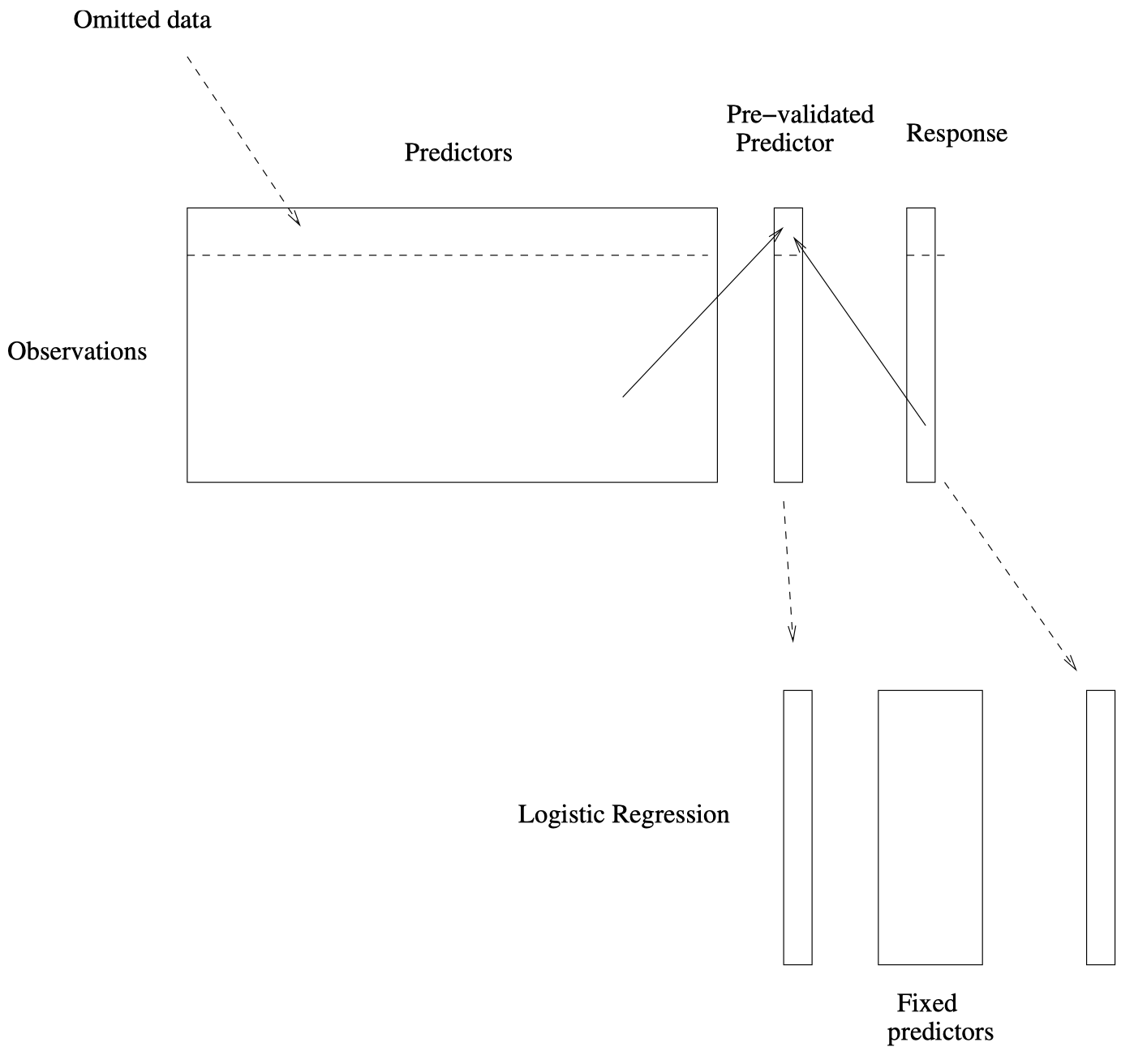1. 将全部 $78$ 个病例分为 $K = 13$ 个相等大小的部分，每部分包含 $6$ 个病例。
2. 留出其中一个部分。仅使用其余 $12$ 个部分的数据，选择与类别标签具有至少 $0.3$ 的绝对相关性的特征，并构建最近质心分类规则。
3. 使用构建好的分类规则预测之前留出部分的类别标签。
4. 对 $13$ 个部分中的每个部分都执行上述步骤 2 和 3，从而为 $78$ 个病例中的每一个都生成一个 “预验证” 微阵列预测变量 $\tilde z_i$。
5. 对生成的预验证微阵列预测变量和其他 6 个临床预测变量拟合一个逻辑回归模型。

### 2.8 Bootstrap vs. 置换检验 (Permutation tests)

• Bootstrap 从 估计总体 (estimated population) 中抽样，并使用其结果来估计 标准误置信区间

• 置换方法从数据的 估计零分布 (estimated null distribution) 中抽样，并用它来估计 p 值 和假设检验的 错误发现率(False Discovery Rates, FDR)

• Bootstrap 可用于在简单情况下 检验零假设。例如，如果 $H_0: \theta=0$，则我们可以检查 $\theta$ 的置信区间中是否包含 $0$。

• 我们也可以将 bootstrap 抽样用于零分布 (请参阅 Efron 和 Tibshirani 的书 《An Introduction to the Bootstrap》(1993, 第 16 章))，但这种做法与置换方法相比并不存在真正的优势。本作品采用知识共享署名-非商业性使用-相同方式共享 4.0 国际许可协议进行许可。 欢迎转载，并请注明来自：YEY 的博客 同时保持文章内容的完整和以上声明信息！